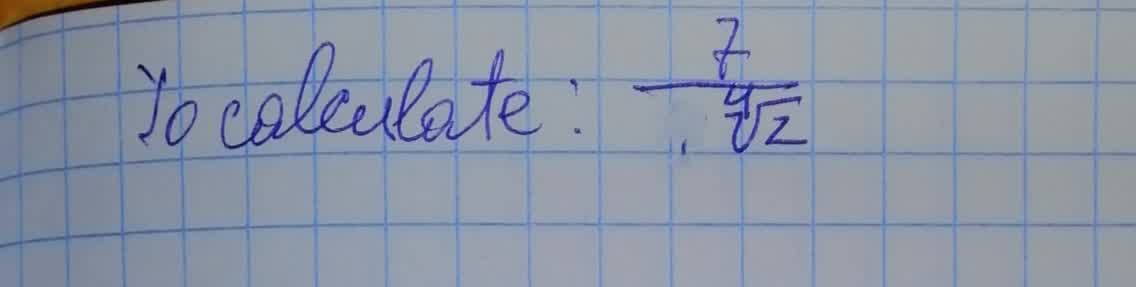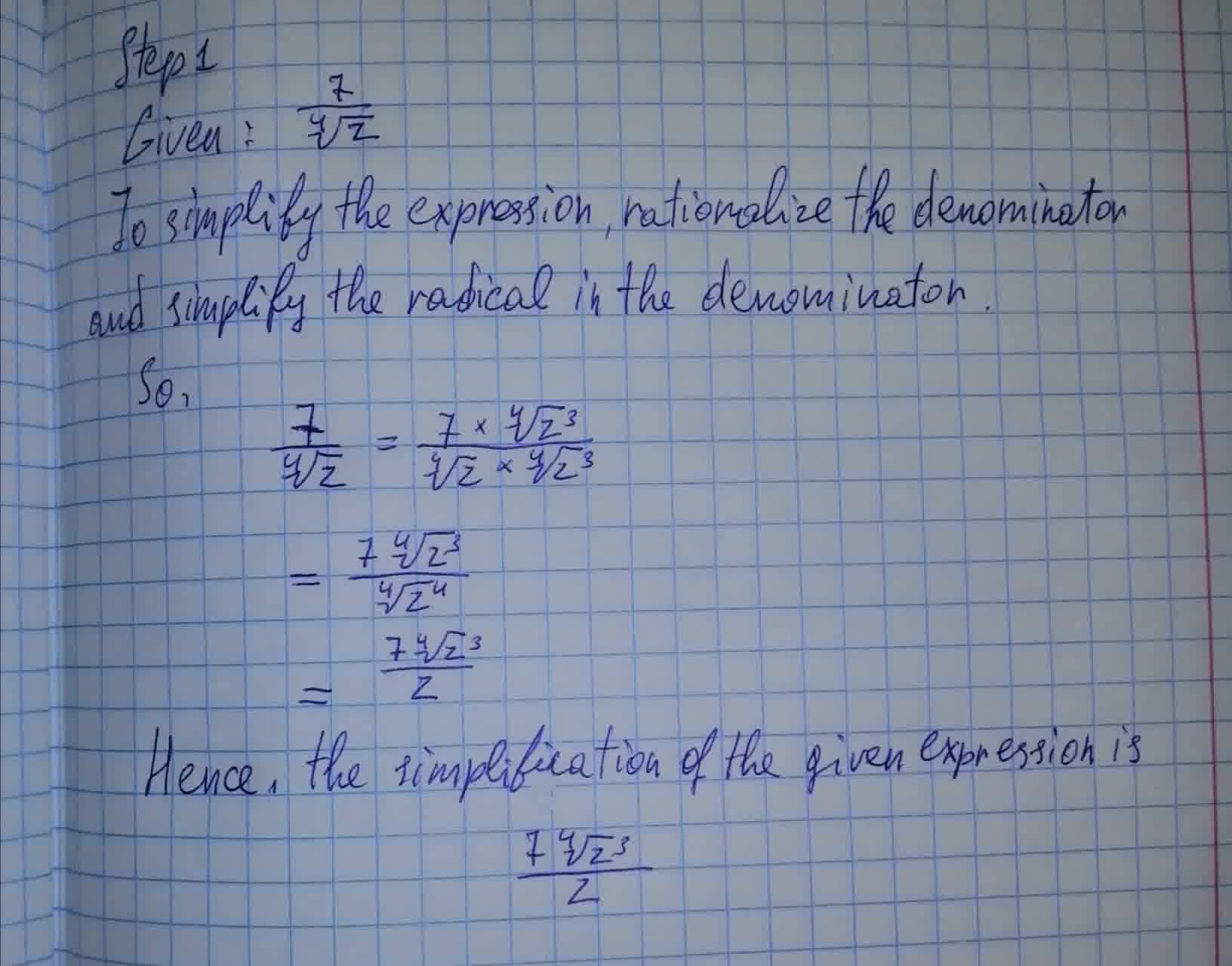To calculate: The simplification of the expression \frac{7}{\sqrt{z}}allhvasstH 2021-08-14 Answered

To calculate: The simplification of the expression $$\frac{7}{\sqrt{z}}$$• Questions are typically answered in as fast as 30 minutes

Solve your problem for the price of one coffee

• Math expert for every subject
• Pay only if we can solve itokomgcae
Now we haveNot exactly what you’re looking for?

Step 1

Given: $$\frac{7}{\sqrt{z}}$$

To simplify the expression, rationalize the denominator and simplify the radical in the denominator

So,

$$\frac{7}{\sqrt{z}}=\frac{7 \times \sqrt{z^3}}{\sqrt{z} \times \sqrt{z^3}}$$

$$=\frac{7\sqrt{z^3}}{\sqrt{z^4}}$$

$$=\frac{7 \sqrt{z^3}}{z}$$

Hence, the simplification of the given expression is

$$\frac{7 \sqrt{z^3}}{z}$$